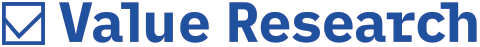# Insights into the expense ratio

##### The NAV of the fund is inclusive of the expense ratio and this is charged in a small proportion each day, says Ashutosh Gupta

Would you please elaborate on the expense ratio? Is this NAV inclusive of the expense ratio? Exactly when are these expenses deducted?
- K Venkata Subbarao

You can consider the expense ratio as the cost that a fund company charges you for the services it is providing. Besides, there could be certain additional costs added to it. For example, the distributor's commission in the case of regular plans. So, to put it in a nutshell, that's what an expense ratio is.

Now, the expense ratio is not charged on a particular day in a year. It is charged on a drip-by-drip basis every single day from the NAV and the NAV that the fund discloses every day is after charging the expense ratio. So, for instance, if a fund is charging 1 per cent of its AUM as the expense ratio, then this 1 per cent is not charged on a particular day, but every single day a very small proportion of this 1 per cent is charged from the NAV and thereafter, the NAV is disclosed.

Beyond NAV, there are a few other nominal charges that you would have to bear. For instance, at the time of buying the fund, you will have to incur a stamp duty which is at a rate of Rs 5 for an investment worth Rs 1 lakh. Likewise, at the time of selling, there is a securities transaction tax that has to be paid, which is at the rate of 0.001 per cent in the case of equity funds. So, those are the pretty nominal charges which sit outside of the expense ratio. Otherwise, the expense ratio factors in your overall ownership costs of mutual fund units. Hence, the returns that you calculate using NAV are the actual returns that you get as the expense ratio has already been factored into while calculating the NAV.